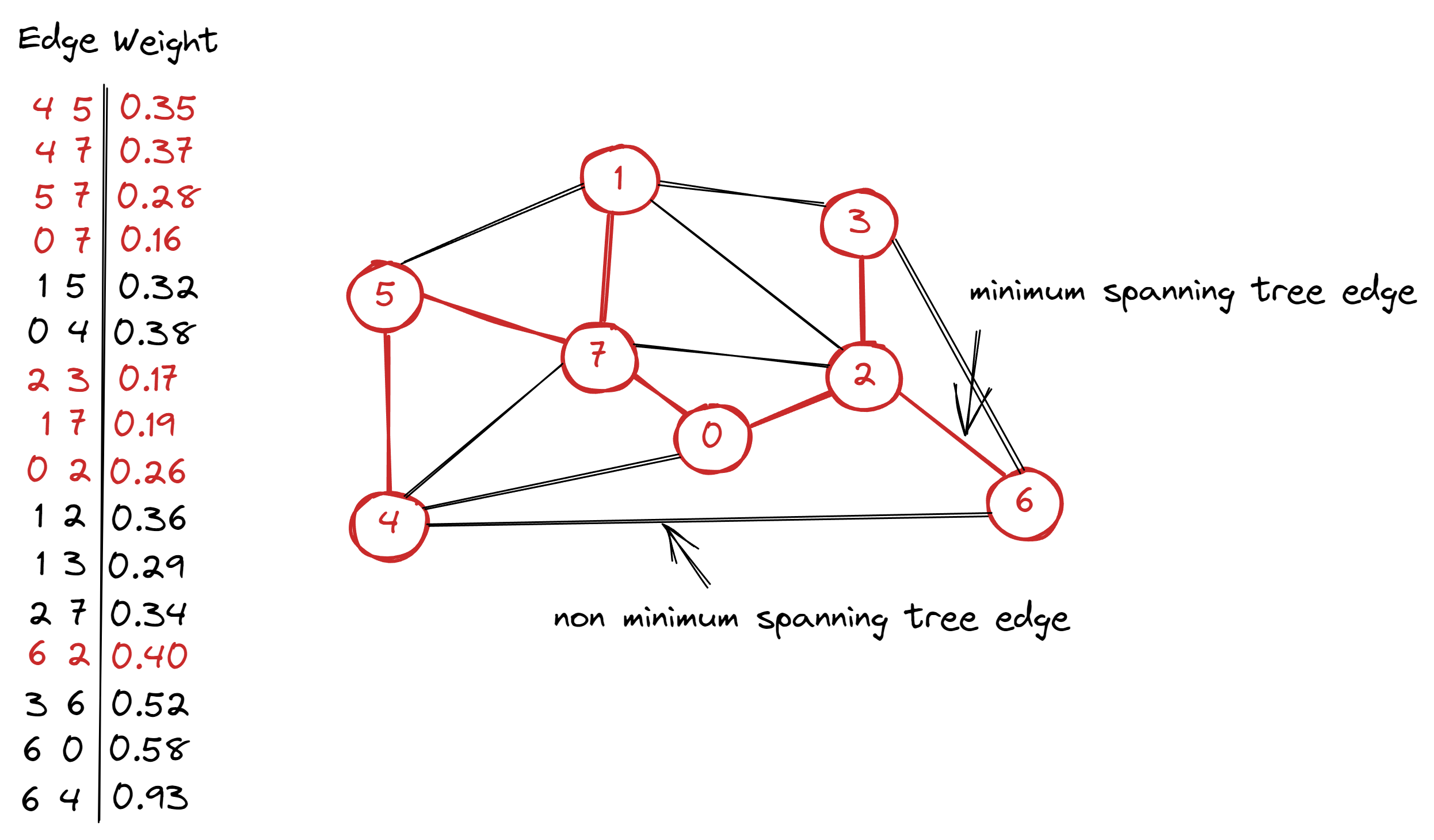savchenko.tech / software engineering blog by Maryna Savchenko## Prim's algorithm. Greedy. Algorithms series

2022-08-18

Prim’s algorithm is a greedy algorithm for computing Minimum Spanning Tree (MST).

Greedy algorithms is a technique used in designing and analyzing efficient algorithms. They typically apply to optimization problems in which you make a set of choices in order to arrive at an optimal solution. A greedy algorithm always makes the choice that looks best at the moment. It makes a locally optimal choice in hope that this choice leads to globally optimal solution.

Minimum spanning tree (MST) of an edge-weighted graph is spanning tree whose weight (the sum of the weights of its edges) is no larger than the weight of any other spanning tree.

Spanning tree of a graph is a connected subgraph that includes all the vertices and has no cycles.Unit test should check that there are right amount of edges in MST and the weight of MST is correct.

``````   @Test
void shouldReturnMSTEdges() {
WeightedGraph weightedGraph = createWeightedGraph();

MinimumSpanningTreePrims mst = new MinimumSpanningTreePrims(weightedGraph);
Queue<Edge> mstEdges = mst.getMstEdges();

assertEquals(7, mstEdges.size());
}

@Test
void shouldReturnRightMSTWeight() {
WeightedGraph weightedGraph = createWeightedGraph();

MinimumSpanningTreePrims mst = new MinimumSpanningTreePrims(weightedGraph);
double epsilon = 0.000001d;

assertEquals(1.80, mst.getWeight(), epsilon);
}
``````

Implementation of the Prim’s algorithm needs WeightedGraph and Edge classes. It can be found here.

The main idea of Prim’s algorithm is to add a new edge to a single growing tree at each step.

• visit vertex, that has not been visited yet (starting from the first vertex)
• mark it as visited
• get all edges for this vertex
• add edge to priority queue (by weight) if it has not visited vertices
• get (remove) lowest-weight edge from priority queue
``````public class MinimumSpanningTreePrims {
private final boolean[] visitedVertices;
private final Queue<Edge> mstEdges;
private final PriorityQueue<Edge> crossingEdges;

public MinimumSpanningTreePrims(WeightedGraph graph) {
crossingEdges = new PriorityQueue<>();
visitedVertices = new boolean[graph.getNumberOfVertices()];

visit(graph, 0);
while (!crossingEdges.isEmpty()) {
Edge edge = crossingEdges.remove();
int oneVertex = edge.getOneVertex();
int otherVertex = edge.getOtherVertex(oneVertex);
if (visitedVertices[oneVertex] && visitedVertices[otherVertex]) continue;
if (!visitedVertices[oneVertex]) visit(graph, oneVertex);
if (!visitedVertices[otherVertex]) visit(graph, otherVertex);
}

}

public Queue<Edge> getMstEdges() {
return mstEdges;
}

public double getWeight() {
double weight = 0.0;
for (Edge edge : getMstEdges()) {
weight += edge.getWeight();
}
return weight;
}

private void visit(WeightedGraph graph, int vertex) {
visitedVertices[vertex] = true;
for (Edge edge : graph.getEdgesFor(vertex)) {
if (!isOtherVertexVisited(edge, vertex)) {
}
}
}

private boolean isOtherVertexVisited(Edge edge, int vertex) {
int otherVertex = edge.getOtherVertex(vertex);
return visitedVertices[otherVertex];
}
}
``````

Complete implementation with test cases can be found on GitHub.

Sources: